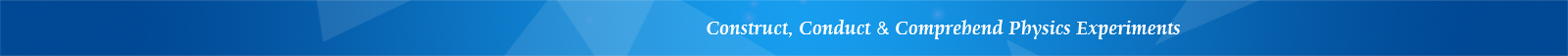## LEMI-37 Comprehensive Experiments on Rotating Liquid

Features

• Resonable in structure design

• Stable and reliable experimental data

• Rich experiment contents

Introduction

The LEMI-37, apparatus of comprehensive experiments on rotating liquid, uses experimental methods such as detecting the inclination of the liquid surface using a semiconductor laser and measuring the rotational period using a Hall sensor. It reproduces the classic rotating liquid experiment based on modern teaching techniques.

The experiment mainly focuses on observing the surface shape of the rotating liquid. The surface shape of the rotating liquid in the cylinder is a parabolic surface whose parameters are related to gravity acceleration, so that gravity acceleration can be derived by measuring the surface shape. The parabolic surface is also a good optical element that can focus a parallel light beam into a spot at its focal plane. This experiment will verify the relationship between the focal length and the rotation period. In addition, the relationship between optical imaging of a liquid concave mirror and rotating speed can also be studied in this experiment. So, the apparatus can perform comprehensive experiments in both subjects of mechanics and optics.

LEMI-37 apparatus of rotating liquid experiments is mainly consists of main electric unit, cylinder container, rotary stage, beam support, Hall sensor, semiconductor laser, observation screen, bubble level, and so on. The apparatus has features of rich in experimental contents, reasonable in structure design, stable and reliable experimental data. It is a high-quality teaching apparatus for physics labs of mechanics and optics subjects in colleges and universities.

Using this apparatus, the following experiments can be conducted:

1. Measure gravity acceleration g using two methods:

(1) measure the height difference between the highest and the lowest points of the surface of rotating liquid, then calculate gravity acceleration g;

(2) laser beam incident parallel to the rotation axis to measure surface slope, then calculate gravity acceleration g;

2. Verify the relationship between focal length f and rotational period T according to the parabolic equation;

3. Study concave mirror imaging of rotating liquid surface.